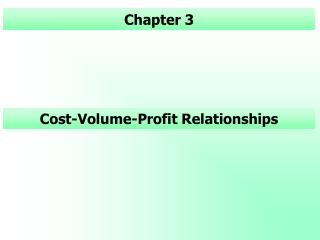# Cost-Volume-Profit Relationships - PowerPoint PPT PresentationDownload PresentationCost-Volume-Profit Relationships

Cost-Volume-Profit Relationships
Download Presentation## Cost-Volume-Profit Relationships

- - - - - - - - - - - - - - - - - - - - - - - - - - - E N D - - - - - - - - - - - - - - - - - - - - - - - - - - -
##### Presentation Transcript

1. Cost-Volume-Profit Relationships Chapter 3

2. Introduction Cost-volume-profit analysis examines the behavior of total revenuestotal costsoperating income as changes occur in the output levelselling pricevariable costs per unit fixed costs.

3. Cost-Volume-Profit Assumptions and Terminology 1 Changes in the level of revenues and costs arise only because of changes in the number of units produced and sold(one revenue driver and one cost driver). Total costs can be divided into a fixed component and a component that is variable with respect to the level of output.

4. Cost-Volume-Profit Assumptions and Terminology 2 The behavior of total revenues and total costs is linear (straight-line) in relation to output units within the relevant range (and time period). The unit selling price, unit variable costs, and fixed costs are known and constant.

5. Cost-Volume-Profit Assumptions and Terminology 3 Assumes a single product or that the sales mix when multiple products are sold will remain constant as the level of total units sold changes. All revenues and costs can be added and compared without taking into account the time value of money.

6. Cost-Volume-Profit Assumptions and Income Definitions Operating income = Total revenues from operations • Cost of goods sold- Operating costs Net Income = Operating income + Non-operating income (e.g. investment income) – Non-operating costs (e.g. such as interest cost) – Income taxes

7. Contribution margin The only numbers that change are total revenues and total variable cost. Contribution margin = Total revenues – Total Variable Costs Unit Contribution Margin = Unit Selling Price – Unit Variable Cost

8. Contribution margin percentage Contribution margin percentage = contribution margin ratio = Unit Contribution Margin ÷ Unit Selling Price

9. Breakeven Point... ;the sales level at which operating income is zero. At the breakeven point, sales revenue minus variable costs equals fixed expenses. Total revenues = Total costs

10. Breakeven Point – Equation Method Operating income = Unit sales price × units sold – Unit Variable Cost × units sold – Fixed costs Operating income + Fixed costs (Unit Sales Price – Unit Variable Cost) × units sold Unit sales needed = (Target Operating income + Fixed costs) ÷ (Unit Sales Price – Unit Variable Cost)

11. Abbreviations USP Unit selling price UVC Unit variable costs UCM Unit contribution margin CM% Contribution margin percentage FC Fixed costs Q Quantity of output (units sold or produced) OI Operating income TOI Target operating income TNI Target net income

12. Contribution Margin Method - Units With the contribution margin method, Unit sales needed is calculated by USP × Q – UVC × Q – FC = OI (USP – UVC) × Q = FC + OI UCM × Q = FC + OI Q = [FC + TOI] ÷ UCM

13. Contribution Margin Method – Dollars \$ sales needed = Q × USP = [FC + OI] ÷ UCM × USP = [FC + OI] ÷ [UCM ÷ USP] = [FC + OI] ÷ Contribution margin ratio Example 1

14. Target Net Income and Income Taxes Tax Rate = TR Unit sales needed is calculated by using the following relationship: [ USP × Q – UVC × Q – FC] × [1-TR] = NI (USP – UVC) × Q = FC + NI/[1-TR] UCM × Q = FC + NI/[1-TR] Q = [FC + NI/(1-TR)] ÷ UCM Target Net Income and Income Taxes

15. Graph Plot a line for total revenues and total costs. The breakeven point is the point at which the total revenue line intersects the total cost line. The area between the two lines to the right of the breakeven point is the profit area.

16. Operating Leverage... ; measures the relationship between a company’s variable and fixed expenses. It is greatest in organizations that have high fixed expenses and low unit variable costs. Operating leverage shows = %change in sales volume ÷ % change in OI Degree of operating leverage = Contribution margin ÷ Operating income Operating Leverage

17. Mix Changes - Titanic Revenue Conrib. CM ratio Theater Plan 440 \$390 0.89 Actual 440 \$396 0.90 Video Plan 576.0 \$395 \$0.68 Actual 518.4 \$358 \$0.69 TV Plan 125 \$125 1.00 Actual 125 \$125 \$1.00 Total Plan 1,141 \$910 \$0.80 Actual 1,083 \$879 \$0.81

18. Contribution MarginversusGross Margin Financial accounting income statement emphasizes gross margin. Revenues – Cost of goods sold = Gross margin – Operating costs = Operating income

19. Financial ManagementAccounting Accounting Revenue Revenue - Cost of Goods Sold: - Variable costs: Variable Production Costs Variable Production costs Fixed Production Costs Variable SG&A costs = Gross Margin = Contribution Margin - SG&A - Fixed Costs Variable SG&A costs Fixed Production Costs Fixed SG&A costs Fixed SG&A costs = Operating Income = Operating Income

20. Income Statement Operating Income + Non Operating Income: Income from affiliates, Interest income ------------------------------ = Earnings Before Interest & Taxes • Interest Expense ------------------------------ = Earnings Before Taxes • Tax Expense ------------------------------ = Net Income